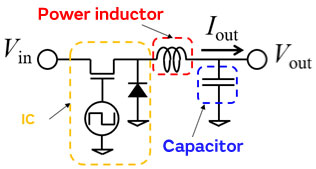# Inductor for Power LinesPower Inductor Basic Course - Chapter 1

## Chapter 1: What is a Power Inductor?

### 1.1 Overview of power inductors

A coil is a generic name for an electrode in the shape of a spiral. Among the different types of coils, there are coils called “inductors” which are used for electrical applications. Inductors can be further categorized into RF inductors used for signal processing, and power inductors for power supply lines. The power inductors discussed in this section form part of the voltage conversion circuit in a DC-DC converter or other device.
Here we will explain the operation of a power inductor in a DC-DC converter. A power inductor is used in a step-up, step-down, or step-up/step-down circuit to convert a certain voltage to the required voltage. Among those different circuits, it is primarily used in a type of circuit called a “switching regulator.”

Figure 1-1 shows an example of a switching regulator step-down circuit.
It uses an IC, power inductor, and capacitor to convert a DC input voltage to the required output voltage. The power inductor works with the capacitor to play the role of rectifying the rectangular wave output from the IC to a direct current (further details are explained in Chapter 2).
If either one of these components is missing, the output cannot be properly rectified.Figure 1-1 Overview of a step-down switching regulator